# Data Structures in Pandas

Pandas is an open-source library that uses for working with relational or labeled data both easily and intuitively. It provides various data structures and operations for manipulating numerical data and time series. It offers a tool for cleaning and processes your data. It is the most popular Python library that is used for data analysis. In this article, We are going to learn about Pandas Data structure.

It supports two data structures:

## Series

Pandas is a one-dimensional labeled array and capable of holding data of any type (integer, string, float, python objects, etc.)

Syntax: pandas.Series(data=None, index=None, dtype=None, name=None, copy=False, fastpath=False)

Parameters:

• data: array- Contains data stored in Series.
• index: array-like or Index (1d)
• dtype: str, numpy.dtype, or ExtensionDtype, optional
• name: str, optional
• copy: bool, default False

Example 1: Series holding the char data type.

## Python3

 `import` `pandas as pd` ` `  `# a simple char list` `list` `=` `[``'g'``, ``'e'``, ``'e'``, ``'k'``, ``'s'``]` `  `  `# create series form a char list` `res ``=` `pd.Series(``list``)` `print``(res)`

Output: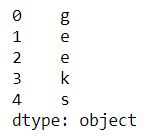Example 2: Series holding the Int data type.

## Python3

 `import` `pandas as pd` ` `  `# a simple int list` `list` `=` `[``1``,``2``,``3``,``4``,``5``]` `  `  `# create series form a int list` `res ``=` `pd.Series(``list``)` `print``(res)`

Output: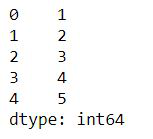Example 3: Series holding the dictionary.

## Python3

 `import` `pandas as pd`   `dic ``=` `{ ``'Id'``: ``1013``, ``'Name'``: ``'MOhe'``, ` `       ``'State'``: ``'Maniput'``,``'Age'``: ``24``}`   `res ``=` `pd.Series(dic)` `print``(res)`

Output: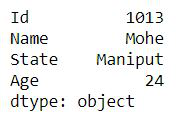## Dataframe

Pandas DataFrame is a two-dimensional size-mutable, potentially heterogeneous tabular data structure with labeled axes (rows and columns). A Data frame is a two-dimensional data structure, i.e., data is aligned in a tabular fashion in rows and columns like a spreadsheet or SQL table, or a dict of Series objects. . Pandas DataFrame consists of three principal components, the data, rows, and columns.

### Creating a Pandas DataFrame

In the real world, a Pandas DataFrame will be created by loading the datasets from existing storage, storage can be SQL Database, CSV file, and Excel file. Pandas DataFrame can be created from the lists, dictionary, and from a list of dictionary etc. Dataframe can be created in different ways here are some ways by which we create a dataframe:

Example 1: DataFrame can be created using a single list or a list of lists.

## Python3

 `# import pandas as pd` `import` `pandas as pd` ` `  `# list of strings` `lst ``=` `[``'Geeks'``, ``'For'``, ``'Geeks'``, ``'is'``, ` `            ``'portal'``, ``'for'``, ``'Geeks'``]` ` `  `# Calling DataFrame constructor on list` `df ``=` `pd.DataFrame(lst)` `display(df)`

Output: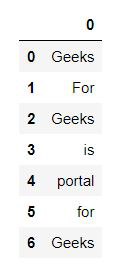Example 2: Creating DataFrame from dict of ndarray/lists.

To create DataFrame from dict of narray/list, all the narray must be of same length. If index is passed then the length index should be equal to the length of arrays. If no index is passed, then by default, index will be range(n) where n is the array length.

## Python3

 `# Python code demonstrate creating ` `# DataFrame from dict narray / lists ` `# By default addresses.` ` `  `import` `pandas as pd` ` `  `# initialise data of lists.` `data ``=` `{``'Name'``:[``'Tom'``, ``'nick'``, ``'krish'``, ``'jack'``],` `        ``'Age'``:[``20``, ``21``, ``19``, ``18``]}` ` `  `# Create DataFrame` `df ``=` `pd.DataFrame(data)` ` `  `# Print the output.` `display(df)`

Output: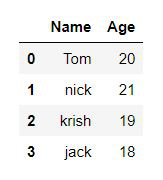### Dealing with a column and row in DataFrame

Selection of column: In Order to select a column in Pandas DataFrame, we can either access the columns by calling them by their columns name.

## Python3

 `# Import pandas package ` `import` `pandas as pd ` `  `  `# Define a dictionary containing employee data ` `data ``=` `{``'Name'``:[``'Jai'``, ``'Princi'``, ``'Gaurav'``, ``'Anuj'``], ` `        ``'Age'``:[``27``, ``24``, ``22``, ``32``], ` `        ``'Address'``:[``'Delhi'``, ``'Kanpur'``, ``'Allahabad'``, ``'Kannauj'``], ` `        ``'Qualification'``:[``'Msc'``, ``'MA'``, ``'MCA'``, ``'Phd'``]} ` `  `  `# Convert the dictionary into DataFrame  ` `df ``=` `pd.DataFrame(data) ` `  `  `# select two columns ` `print``(df[[``'Name'``, ``'Qualification'``]])`

Output: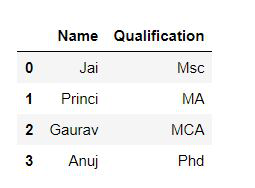### How to Select Rows and Column from Pandas DataFrame?

Example 1: Selecting rows.

pandas.DataFrame.loc is a function used to select rows from Pandas DataFrame based on the condition provided.

Syntax: df.loc[df[‘cname’] ‘condition’]

Parameters:

• df: represents data frame
• cname: represents column name
• condition: represents condition on which rows has to be selected

## Python3

 `# Importing pandas as pd ` `from` `pandas ``import` `DataFrame ` `  `  `# Creating a data frame ` `Data ``=` `{``'Name'``: [``'Mohe'``, ``'Shyni'``, ``'Parul'``, ``'Sam'``], ` `        ``'ID'``: [``12``, ``43``, ``54``, ``32``], ` `        ``'Place'``: [``'Delhi'``, ``'Kochi'``, ``'Pune'``, ``'Patna'``] ` `       ``} ` `  `  `df ``=` `DataFrame(Data, columns ``=` `[``'Name'``, ``'ID'``, ``'Place'``]) ` `  `  `# Print original data frame ` `print``(``"Original data frame:\n"``) ` `display(df) ` `  `  `# Selecting the product of Electronic Type ` `select_prod ``=` `df.loc[df[``'Name'``] ``=``=` `'Mohe'``] ` `  `  `print``(``"\n"``) ` `  `  `# Print selected rows based on the condition ` `print``(``"Selecting rows:\n"``) ` `display (select_prod)`

Output: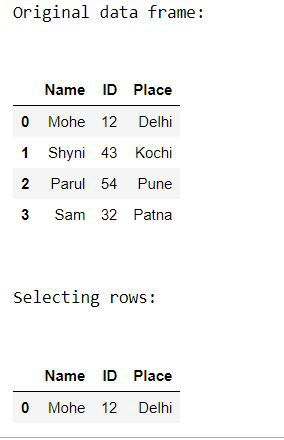Example 2: Selecting column.

## Python3

 `# Importing pandas as pd ` `from` `pandas ``import` `DataFrame ` `  `  `# Creating a data frame ` `Data ``=` `{``'Name'``: [``'Mohe'``, ``'Shyni'``, ``'Parul'``, ``'Sam'``], ` `        ``'ID'``: [``12``, ``43``, ``54``, ``32``], ` `        ``'Place'``: [``'Delhi'``, ``'Kochi'``, ``'Pune'``, ``'Patna'``] ` `       ``} ` `  `  `df ``=` `DataFrame(Data, columns ``=` `[``'Name'``, ``'ID'``, ``'Place'``]) ` `  `  `# Print original data frame ` `print``(``"Original data frame:"``) ` `display(df) ` `  `  `print``(``"Selected column: "``)` `display(df[[``'Name'``, ``'ID'``]] )`

Output: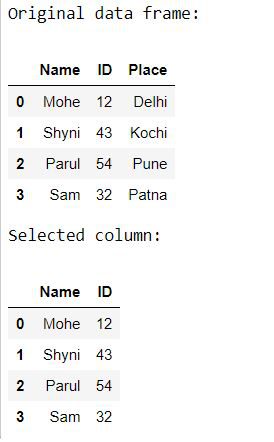Whether you're preparing for your first job interview or aiming to upskill in this ever-evolving tech landscape, GeeksforGeeks Courses are your key to success. We provide top-quality content at affordable prices, all geared towards accelerating your growth in a time-bound manner. Join the millions we've already empowered, and we're here to do the same for you. Don't miss out - check it out now!